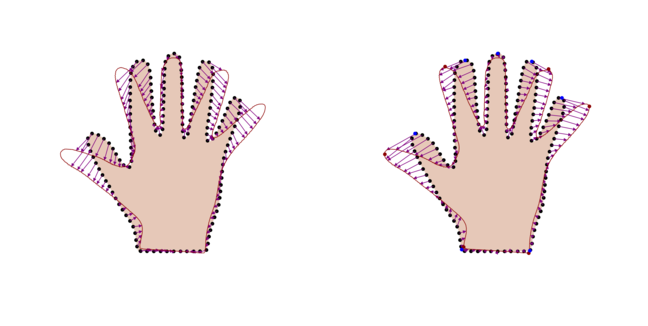## Want to keep learning?

This content is taken from the University of Basel's online course, Statistical Shape Modelling: Computing the Human Anatomy. Join the course to learn more.
6.2

# Model fitting and the registration problem

We have seen that model fitting is an important first step in analysing new shapes by comparing them with the shape model. In this article, we show that the problem of fitting a model to a new shape arises also in the process of model building itself, namely when correspondence has to be established between the example shapes. In this context, model fitting is known as registration. Here, we will discuss the difference between registration and model fitting, briefly sketch the most common registration approach and point the interested reader to further literature.

## Model building revisited

The key idea in statistical shape modelling is to estimate a distribution for the shape deformations from a set of example shapes $$\Gamma_1, \ldots, \Gamma_n$$. So far we assumed that we are given a reference shape $$\Gamma_R$$ and a set of deformations fields $$u_1, \ldots, u_n$$, where each deformation field $$u_i : \Gamma_R \to \mathbb{R}^2$$ defines a mapping between every point of the reference shape $$x \in \Gamma_R$$ and the corresponding point $$x + u_i(x) \in\Gamma_i$$. This allowed us to estimate the shape variations by means of a Gaussian Process defined by the sample mean and sample covariance of these deformation fields.

In practice, however, the target shapes $$\Gamma_1, \ldots, \Gamma_n$$, are usually not in correspondence with each other and the deformation fields cannot be easily calculated. The remaining step to complete our toolchain for shape modelling is therefore the computation of these deformation fields. To this end, we select one of the $$\Gamma_1, \ldots, \Gamma_n$$ to be the reference shape $$\Gamma_R$$. We then seek a deformation field $$u_i : \Gamma_R \to \mathbb{R}^2$$ between the reference and the example shape $$\Gamma_i$$, which is not in correspondence. This is the very problem that can be solved with the Iterative Closest Point (ICP) algorithm.

In Week 4, we learned how to define Gaussian Process models that model shape deformations without resorting to example data. Using such a model in combination with the standard ICP algorithm allows us to find the deformation field. Although the algorithm works unchanged with such generic smooth deformation models, it becomes less robust in general compared to statistical deformation models, because the model no longer enforces that only hand shapes are represented. Figure 1 (left) shows the result of applying the ICP algorithm using a simple Gaussian kernel to model smooth deformations. You can see easily that the algorithm is unable to successfully establish correspondence at the fingertips. The situation is improved by including known deformations into the model by means of Gaussian Process regression and the resulting posterior model. Figure 1 (right) shows the result when the deformations at the given landmark points (the red and blue points) were used to build aposterior model, which was then used in the algorithm.Figure 1: comparison of exemplar results of the ICP algorithm used with a model of smooth deformations. Left: using only a model of smooth deformation, the correct deformation at the fingertips cannot be found. Right: by using a posterior model that includes the deformation given by the landmarks (red and blue points) a much better solution is obtained.

## Model fitting and registration

We have now seen two different applications of model fitting. In the first one, a statistical shape model is fitted to a target shape in order to analyse this shape using the model. In the second application, model fitting is used to build the model itself. In this latter application, model fitting was used to find a solution to the registration problem, which is the problem of establishing correspondence between two shapes.

In the literature, registration and model fitting have usually been treated as two separate problems. Instead of being treated as the task of fitting a model of smooth deformations, registration is usually formulated as an optimisation problem

$$\DeclareMathOperator{\argmin}{arg\,min} u^* = \argmin_u D[\Gamma_R, \Gamma_T, u] + \eta R[u],$$

where $$D$$ is a distance measure between two surfaces, $$R$$ a regularisation term and $$\eta \in \mathbb{R}$$ a weight factor. In this alternative approach, the regularisation term $$R$$ encodes the prior assumption. It penalises deformations that do not match the encoded assumption. It turns out that many popular regularisation approaches can in fact be formulated as a Gaussian Process model.

## Further explorations into registration

A detailed discussion of registration would go beyond the scope of this course. We refer the interested reader to the papers  and  for a more detailed introduction to surface and image registration respectively.

We also highly recommend the paper by Steinke et al.  that explains the connection between the regularisation approach and the Gaussian Process view in an easy and accessible way, using only concepts from linear algebra.

As a final remark, we would like to point out that the general approach to the registration problem outlined above is implemented in Scalismo and can be used for both surface and image registration. The quickstart guide that you can find in Scalismo Lab explains in detail how this registration framework can be used for surface registration.